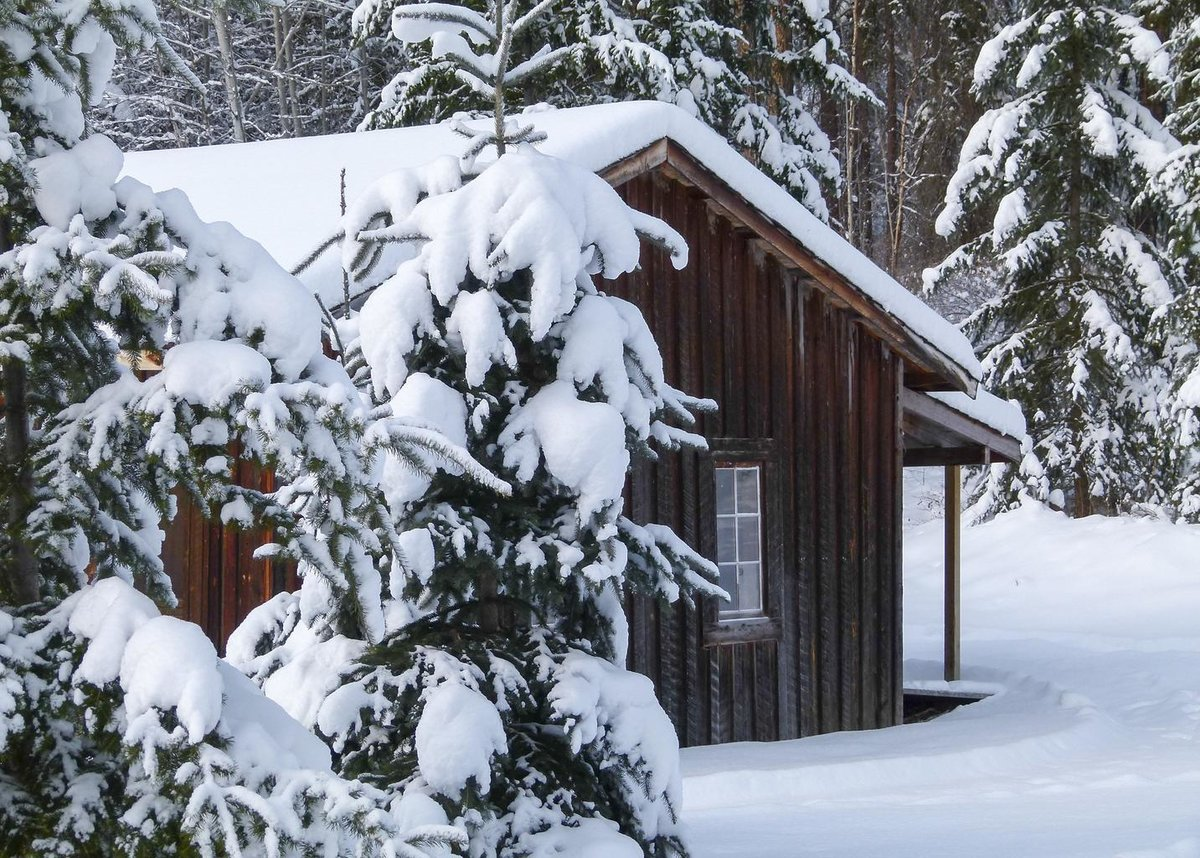# How to Blend Multiple Images Using OpenCVIn this project, we will blend multiple images using OpenCV. “Blending” means that we compute a weighted average of the pixel values for a set of color images which have the same dimensions.

# You Will Need

• Python 3.7+
• A bunch of images that you want to blend together.

# Directions

Let’s say you have a set of images. You would like to create a new image which is the average of all the images.

For example, I have about 10 images which I obtained from this weather forecast website. I’m interested in seeing the areas which will receive the most snow on average over the next 10 days (i.e. the darkest blues). In order to do that I need to create a single image which blends the weather forecast frames for the next 10 days.

Below is a slide show of the images I would like to blend.

Let’s blend all those images so that we create an “average” image. Here is the code:

```# Python program for blending multiple images using OpenCV

import glob
import numpy as np
import cv2

# Import all image files with the .jpg extension
files = glob.glob ("*.jpg")
image_data = []
for my_file in files:
image_data.append(this_image)

# Calculate blended image
dst = image_data
for i in range(len(image_data)):
if i == 0:
pass
else:
alpha = 1.0/(i + 1)
beta = 1.0 - alpha
dst = cv2.addWeighted(image_data[i], alpha, dst, beta, 0.0)

# Save blended image
cv2.imwrite('weather_forecast.png', dst)
```

What I’m doing above is importing all images in the current directory that have the .jpg extension.

I then put each image into a list.

I multiply each image by a weight. The weight depends on how many images there are. So for example, if I have 10 images in total, each image gets multiplied by 1/10.

After computing the “average” image, I save it as weather_forecast.png. Here is the result:

Pretty cool! We can see that the snowiest areas will be in Utah, central Arizona, and southwest portions of Colorado. Now I know where to hit the slopes!

Keep building!